# KR20120054879A - Method for planing collision avoidance path of car-like mobile robot and parking assist system using the same - Google Patents

Method for planing collision avoidance path of car-like mobile robot and parking assist system using the same Download PDF

## Info

Publication number
KR20120054879A
KR20120054879A KR1020100116244A KR20100116244A KR20120054879A KR 20120054879 A KR20120054879 A KR 20120054879A KR 1020100116244 A KR1020100116244 A KR 1020100116244A KR 20100116244 A KR20100116244 A KR 20100116244A KR 20120054879 A KR20120054879 A KR 20120054879A
Authority
KR
South Korea
Prior art keywords
motion
path
mobile robot
set
vehicle
Prior art date
Application number
KR1020100116244A
Other languages
Korean (ko)
Inventor
권현기
김달형
정우진
Original Assignee
고려대학교 산학협력단
Priority date (The priority date is an assumption and is not a legal conclusion. Google has not performed a legal analysis and makes no representation as to the accuracy of the date listed.)
Filing date
Publication date
Application filed by 고려대학교 산학협력단 filed Critical 고려대학교 산학협력단
Priority to KR1020100116244A priority Critical patent/KR20120054879A/en
Publication of KR20120054879A publication Critical patent/KR20120054879A/en

• 230000000875 corresponding Effects 0 claims abstract description 20
• 238000006073 displacement Methods 0 claims abstract description 16
• 238000004364 calculation methods Methods 0 claims description 25
• 238000004088 simulation Methods 0 description 23
• 238000000034 methods Methods 0 description 16
• 239000000203 mixtures Substances 0 description 13
• 238000006243 chemical reaction Methods 0 description 5
• 230000000694 effects Effects 0 description 3
• 238000005516 engineering processes Methods 0 description 2
• 230000001276 controlling effects Effects 0 description 1

## Images

•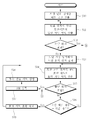•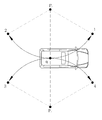•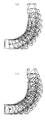•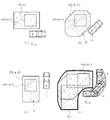•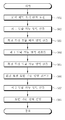•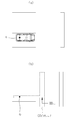•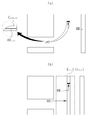•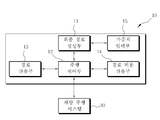•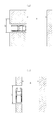•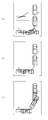•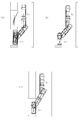•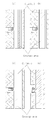•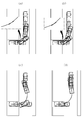•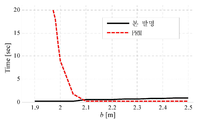## Abstract

According to an aspect of the present invention, there is provided a method of generating a collision avoidance path of a vehicle type mobile robot, comprising: (a) calculating a direction unit obstacle coordination space with respect to an area where a pose and an obstacle of the vehicle type mobile robot overlap with each other in a preset direction angle displacement unit; (b) a reachable area according to at least one motion set from a target position of the vehicular mobile robot is calculated based on the direct unit obstacle coordination space, wherein the plurality of motion sets are continuously connected to form a continuous path Calculating the reachable area in units of the respective motion sets; (c) if the reachable area of each motion set includes a start position of the vehicular mobile robot, setting the reachable area including the start position as a start allowance area; (d) a candidate path set having at least one candidate path from the starting position to the arrival position via backpropagation based on a combination of one or successive motion sets comprising the motion set corresponding to the starting allowance area; Generated; (e) generating one of the plurality of candidate paths as a final path. Accordingly, the generality that can be applied in any environment in the path generation of the vehicle-type mobile robot, the simplicity and the optimality that are low in computational cost and the path is not complicated Can be provided.

## Description

Method for creating collision avoidance path of vehicle-type mobile robot and parking assistance system using the same {METHOD FOR PLANING COLLISION AVOIDANCE PATH OF CAR-LIKE MOBILE ROBOT AND PARKING ASSIST SYSTEM USING THE SAME}

The present invention relates to a collision avoidance path generation method of a vehicle type mobile robot and a parking assistance system using the same. More specifically, the generality which can be applied in any environment in the path generation and the calculation cost for the path generation are described. This invention relates to a collision avoidance path generation method for a vehicle-type mobile robot capable of generating a path that can guarantee low simplicity and optimality, and a parking assistance system using the same.

Recently, various intelligent functions are applied to an automated vehicle, and one of them is a parking assist system. When using this parking assistance system, if the driver selects the parking assistance mode, the target parking lot is scanned by a sensor such as an on-board range sensor.

Then, when the driver moves the vehicle to the initial position, the parking assistance system automatically calculates the main surface environmental terrain and the target parking area from the sensor information. The parking assistance system then generates a target trajectory for parking and controls the control for moving the vehicle to the target parking zone, for example Electric Power Steering (EPS).

On the other hand, among the mobile robots that drive automatically, the car-like mobile robot (Car-like mobile robot), that is to say the path planning for the vehicle-type mobile robot that has four wheels and rotates according to the steering of the front wheel like a conventional vehicle Many studies have been ongoing.

One route planning method for vehicle type mobile robots is to use chained form conversion with sinusoidal input or polynomial input.

Methods using sinusoidal inputs are described in S. Sekhavat and J.-P. Laumond's paper, Topological property for Collision free Nonholonomic Motion Planning: The Case of Sinusoidal Inputs for Chained Form Systems (IEEE Trans. On Robotics and Automation, 1998, 14, (5), pp. 671-680), and RM Murray And SS Sastry's paper, 'Nonholonomic Motion Planning: Steering Using Sinusoids (IEEE Trans. On Automatic Control, 1993, 38, (5), pp. 700-716)', and a method using polynomial input is described in D. Tilbury, R. Murray and S. Sastry's paper, `` Trajectory generation for the n-trailer problem using Goursat normal form (IEEE Trans. On Automatic Control, 1995, 40, (5), pp. 802-819) ''. have. Here, in the method using the chained form conversion, the input is easily calculated by solving the algebraic equation given the boundary condition.

In addition, Kang-Zhi Liu, Minh Quan Dao and Takuya Inoue's paper 'An Exponentially ε-Convergent Control Algorithm for Chained Systems and Its Application to Automatic Parking Systems (IEEE Trans.on Control Systems Technology, 2006, 14, (6), In pp. 1113-1126, an automatic parking system based on chained form conversion using an auxiliary point was proposed.

However, even if chained form conversion is suitable for higher order under-actuated systems, there are problems with many limitations on parking path planning, typically due to nonlinear conversion equations, making collision checks difficult, and sometimes the final path There is a problem of being very repetitive.

Meanwhile, in JAReed and RAShepp's paper 'Optimal paths for a car that goes both forward and backwards' (Pacific Journal of Mathematics, 1990, 145, (2), pp. 367-393), The theory is that a short viable path is a continuation of straight lines and curves. In this paper, 48 possible motion sets are disclosed that connect two configurations. However, the method disclosed in the papers of J.A.Reed and R.A.Shepp is difficult to apply to the parking control of a vehicle type mobile robot because the method disclosed in this paper focuses on an obstacle-free environment.

JPLaumond, PEJacobs, M.Taix, and RMMurray's article, A motion planner for nonholonomic mobile robots (IEEE Trans.Robotics and Automation, 1994, 10, (5), pp. 577-593) A (2-step) approach is disclosed. In the first phase, a collision-free path between two coordinations is planned without consideration of non-holonomic constraints. In the second step, the collision-free path is approximated as a feasible non-holographic path. However, this two-step approach has a problem in that it is not easy to approximate the holographic path by a smooth non-holographic path in a cluttered environment. Therefore, when the vehicle-type mobile robot travels in the lateral direction, there is a problem that the non-holographic path causes a lot of movement.

In addition, J. Barrquand and JCLatombe's paper, 'Nonholonomic multibody mobile robots: controllability and motion planning in the presence of obstacles (Algorithmica, Springer Verlag, 1993, 10, (2), pp. 121-155)' Disclosed are a non-holographic motion planning method based on exhaustive search and several heuristics. However, since the C-space disclosed in this paper is discretized in a grid map, accuracy is dependent on grid resolution. Therefore, the method using C-space provides the biggest disadvantage, even though it provides a practical solution in various cases.

Recently, Kavraki.LE, Svestka.P, Latombe.J.-C, and Overmars' Probabilistic Roadmaps for Path Planning in High Dimensional Configuration Spaces (IEEE Trans. On Robotics and Automation, 1996, 12, (4), pp 566-580) or by NAMelchior and R. Simmons, Particle RRT for Path Planning with Uncertainty (Proc. Int. Conf. Robotics and Automation, Roma, Italy, April, 2007, pp. 1617-1624). The disclosed Probabilistic Roadmap (PRM) planning method is a well known method for the path planning of a mobile robot. The PRM planning method allocates many sample points to the C-space and suggests that the roadmap graph is constructed by linking the sample points. In general, the PRM planning method is useful for dealing with complex robotic systems in complex environments, and its usefulness in parking control is also recognized. However, there is a problem that the PRM planning method does not provide a solution in a narrow passage.

In addition to the path planning technique described above, there have been various attempts related to the automatic parking of vehicles, but the conventional vehicle parking control methods, such as fuzzy control and neural network control, are designed from the perspective of soft computing technology, It can be easily applied, but it lacks the generality of environmental changes.

Accordingly, the present invention has been made to solve the above problems, the generality that can be applied in any environment in the path generation of the vehicle-type mobile robot (Generality), the calculation cost for the path generation is low and the path is An object of the present invention is to provide a collision avoidance path generation method for a vehicle-type mobile robot capable of providing uncomplicated simplicity and optimality, and a parking assistance system using the same.

According to the present invention, in the collision avoidance path generation method of the vehicle-type mobile robot, (a) the orientation unit obstacle coordination space for the area where the pose and the obstacle of the vehicle-type mobile robot overlaps in a preset direction angle displacement unit Calculating a; (b) a reachable area according to at least one motion set from a target position of the vehicular mobile robot is calculated based on the direct unit obstacle coordination space, wherein the plurality of motion sets are continuously connected to form a continuous path Calculating the reachable area in units of the respective motion sets; (c) if the reachable area of each motion set includes a start position of the vehicular mobile robot, setting the reachable area including the start position as a start allowance area; (d) a candidate path set having at least one candidate path from the starting position to the arrival position via backpropagation based on a combination of one or successive motion sets comprising the motion set corresponding to the starting allowance area; Generated; (e) is achieved by a collision avoidance path generation method of a vehicular mobile robot, characterized in that any one of the plurality of candidate paths is generated as a final path.

Wherein the motion set is set such that at least one straight motion and at least one rotational motion are performed continuously in a preset order; (f) the steps (b), (c), and (e) are repeatedly performed according to a change of at least one of the directional angular displacement of the rotational motion and the straight distance of the straight motion to generate a plurality of candidate paths. Further comprising generating a set; In the step (e), any one of the plurality of candidate paths constituting the plurality of candidate path sets may be determined as the final path.

In addition, one of the motion sets may be set in order of straight motion, rotational motion, and straight motion.

In addition, the rotation motion may be set to rotate at the maximum steering angle of the vehicle-type mobile robot.

In addition, in the step (a), the directional unit obstacle coordination space may be calculated by a Minkowski sum between the occupied area according to the appearance of the vehicle-type mobile robot and the occupied area of the obstacle.

Here, in step (a), the occupying area of the obstacle may be applied to the Minkowski sum in a state in which it is expanded to a preset extension size.

And, in the step (b), the reachable area for the one motion set is (b1) the initial position of the motion set of the vehicle-type mobile robot is set; (b2) there is a first reachable region for the first straight motion constituting the motion set based on the orientation unit obstacle coordination space corresponding to the motion initial orientation angle of the vehicular mobile robot at the motion set initial position; Calculated steps; (b3) calculating a rotational motion impingement free coordination region with respect to the rotational angle of the rotational motion constituting the mosen set based on at least one directing unit obstacle coordination space; (b4) shifting the first reachable area according to a position deviation between a preset motion reference point and a robot reference point of the vehicle-type mobile robot at an initial rotational position of the vehicle-type mobile robot in the rotational motion; Wow; (b5) calculating an intersection region between the rotational motion outgoing free configuration region and the shifted first reachable region as a rotational motion reachable region; (b6) shifting the rotational motion reachable area according to a positional deviation between the robot reference point and the motion reference point at the final rotational position of the rotational motion; (b7) a second straight line constituting the motion set based on the directional unit obstacle coordination space and the shifted rotational motion reachable area corresponding to the motion final orientation angle of the vehicular mobile robot at the rotational final position; Calculating a second reachable region for the motion; (b8) may be calculated by performing the step of setting the second reachable region as the reachable region for the corresponding motion set.

In the step (b1), when the calculation of the reachable region for the motion set is started from the target position, the target position is set to the motion set initial position; In the case of the combination of the continuous motion sets, one position selected within the reachable area calculated for the previous motion set may be set as the motion set initial position.

The step (b3) may include: (b31) calculating a trajectory pose in the trajectory of the rotational motion in the direction of the angular displacement based on the rotational angle of the rotational motion; (b32) shifting the orientation unit obstacle coordination space corresponding to the orientation angle of the vehicle-type mobile robot in each trajectory pose according to a positional deviation between the motion reference point and the robot reference point in each trajectory pose Wow; (b33) calculating a rotation motion obstacle coordination region for the rotational motion according to the combination of the shifted direct unit obstacle coordination spaces calculated for each trajectory pose; (b34) The rotation motion collision free configuration region may be calculated based on the rotation motion obstacle configuration region.

And (b2), (b22) calculating a directional angle collision free coordination region at the motion initial oriented angle based on the directional unit obstacle coordination space corresponding to the motion initial oriented angle; (b23) calculating the first reachable region according to a trajectory extension from the motion set initial position to the motion initial orientation angle direction within the directivity angular collision free coordination region.

(G) When the number of the motion sets continuously connected in the step (b) reaches a preset reference number, the combination of the corresponding mossen sets may be deleted in the generation of the candidate path set.

Wherein step (e) comprises: (e1) extracting a plurality of path determination values from each candidate path; (e2) calculating path costs for each candidate path based on the respective path determination values and weights assigned to the respective path determination values; (e3) determining the final path based on the path cost calculated for each candidate path.

The path determination value may include a minimum distance between the vehicle type mobile robot and the obstacle in the candidate path; A change count of the steering of the vehicular mobile robot on the candidate path; Number of turnovers between forward and backward on the candidate path; It may include at least two or more of the moving distance of the vehicle-type mobile robot in the candidate path.

On the other hand, according to another embodiment of the present invention, the final path generation unit for generating a final path in accordance with the collision avoidance path generation method of the vehicle-type mobile robot; It can also be achieved by the parking assistance system, characterized in that it comprises a driving control unit for controlling the driving of the vehicle based on the final path generated by the final path generation unit.

The apparatus may further include a path selection unit for selecting any one of a plurality of candidate paths generated according to the collision avoidance path generation method of the vehicle type mobile robot; The driving controller may control the final path generator to generate the candidate path selected by the path selector as the final path.

The apparatus may further include a path cost calculator configured to calculate path costs for a plurality of candidate paths generated according to the collision avoidance path generation method of the vehicle type mobile robot; The path cost calculation unit extracts a plurality of path determination values from each candidate path, and calculates the path cost for each candidate path based on the respective path determination values and the weights assigned to the path determination values. Yield; The final path generation unit may select the final path based on the path cost calculated for each candidate path.

The path determination value may include a minimum distance between the vehicle type mobile robot and the obstacle in the candidate path; A change count of the steering of the vehicular mobile robot on the candidate path; Number of turnovers between forward and backward on the candidate path; It may include at least two or more of the moving distance of the vehicle-type mobile robot in the candidate path.

Wherein the apparatus further includes a weight input unit for inputting the weight value for each path determination value; The driving controller may control the route generation unit to generate the final route based on the weight inputted through the weight input unit.

Through the above configuration, the generality that can be applied in any environment in the path generation of the vehicle type mobile robot, the simplicity of which the calculation cost for the path generation is low, the path is not complicated, and the optimal Provided are a collision avoidance path generation method for a vehicle-type mobile robot capable of providing optimality and a parking assistance system using the same.

1 is a view for explaining a collision avoidance path generation method of a vehicle-type mobile robot according to the present invention,
2 is a view showing an example of a rotational motion applied to the collision avoidance path generation method of a vehicle-type mobile robot according to the present invention,
3 and 4 are views for explaining the calculation process of the rotational motion obstacle coordination region in the collision avoidance path generation method of the vehicle-type mobile robot according to the present invention,
5 to 7 are views for explaining a method for calculating the reachable region for one motion set in the collision avoidance path generation method of a vehicle type mobile robot according to the present invention,
8 is a view showing the configuration of a parking assistance system according to the present invention,
9 to 14 are views for explaining the effect of the collision avoidance path generation method of the vehicle-type mobile robot according to the present invention.

Hereinafter, with reference to the accompanying drawings will be described in detail the present invention.

1 is a view for explaining a collision avoidance path generation method of a vehicle-type mobile robot according to the present invention.

Referring to FIG. 1, first, the directional unit obstacle coordination space is calculated (S10). Here, T.Lozano-Perez's paper 'Spatial planning: a configuration space approach (Proc. Int. Conf. Computers, May 1983, vol. 32, pp. 108-120) is used to calculate the directional unit obstacle coordination space according to the present invention. The concept of configuration space disclosed in ')' is applied. More specifically, the configuration of the vehicle-type mobile robot is composed of x, y, which are coordinates with respect to the target position, and the orientation angle θ of the vehicle-type mobile robot. And, a set of all coordinations, including vehicle-type mobile robots and obstacles, forms the coordination spaces defined in the paper.

The obstacle coordination space CO becomes a coordination set for obstacles overlapping the movement of the vehicle-type mobile robot, and the direct unit obstacle coordination space is a coordination space at the orientation angle θ of the vehicle-type mobile robot, that is, CO (θ). In addition, all collision free coordination forms a collision free configuration space C free , and the collision free coordination space at the orientation angle θ of the vehicle-type mobile robot can be expressed as C free (θ).

The position of the vehicle-type mobile robot is defined as one point q, that is, one point on (x, y) coordinates. In the present invention, the point q indicating the position of the vehicle-type mobile robot is defined as a robot reference point.

Meanwhile, the directing unit obstacle coordination space according to the present invention is calculated in a preset directing angle displacement unit. More specifically, when setting the directional angular displacement in units of π / 4, the directional unit obstacle coordination space is calculated in units of 0, ± π / 4, ± π / 2, ± 3 π / 4, and 2 π. That is, CO (0), CO (± π / 4), CO (± π / 2), CO (± 3π / 4) and CO (2π) are calculated as the direct unit obstacle coordination space.

In the present invention, it is assumed that the direct unit obstacle coordination space is calculated by the Minkowski sum between the occupied area according to the appearance of the vehicle-type mobile robot and the occupied area of the obstacle. In addition, the occupied area of the obstacle is applied to the Minkowski sum in a state of being expanded to a preset extension size, thereby ensuring stability against collisions.

As such, the direct unit obstacle coordination space CO (θ) for obstacles in the traveling environment of the vehicle-type mobile robot is calculated in the preprocessing step in each directing angular displacement unit, that is, in the preprocessing step before the process of finding a substantial candidate path to be described later. It is possible to effectively reduce the overall computational cost for path generation.

When the direct unit obstacle coordination space CO (θ) is calculated through the above method, the candidate path search process is performed by calculating the reachable region for the motion set.

Herein, the motion set according to the present invention is set such that at least one straight motion and at least one rotational motion are continuously performed in a predetermined order, and in the present invention, one motion set is a sequence of straight motion, rotational motion, and straight motion. For example, it is set to a sequential combination of three motions. In addition, the rotational motion is set to rotate to the maximum steering angle of the vehicle-type mobile robot as an example.

In this way, the motion set is set to the motion pattern of one motion set in the order of straight motion, rotational motion, and straight motion, and the rotational motion is also set to rotate at the maximum steering angle of the vehicle-type mobile robot, so that the calculation cost required for the path search is achieved. Can be significantly reduced.

Here, when the direction angle θ of the vehicle-type mobile robot is given, the reachable area of the straight motion at the direction angle θ can be calculated by expansion in the collision free coordination space C free (θ) with respect to the direction angle θ, Detailed description thereof will be described later.

Rotational motion occurs under maximum steering angle as described above. The rotational motion requires the orientation angle θ start in the starting pose of the vehicle type mobile robot, the orientation angle θ final in the final pose, and the rotation direction. Here, the rotation direction of the rotational motion may be determined by the straight line speed v and the steering angle φ. As described above, in the present invention, since the steering angle is set to the maximum steering angle, the input for the rotational motion is illustrated in FIG. 2. As shown, one of four combinations of [v, -v] x [-? Max,? Max] becomes selectable. 1, 2, 3, and 4 in FIG. 2 represent four directions of rotational motion, and Pr represents a rotational center.

In the present invention, in order to determine an effective algorithm for collision check, it is assumed that the boundary of the obstacle is extended in consideration of the swept area for the motion of the vehicle-type mobile robot. This expansion of the obstacle boundary is useful for the rotational motion of the vehicle type mobile robot.

In this regard, the paper discloses a slice projection technique, which applies a polygonal approximation of the swept region. Therefore, the final performance is affected by the approximation technique, the shape of the robot, and the environmental topography. In the present invention, generality is achieved by using a simple continuation of the direct unit obstacle coordination space CO (θ) without the assumption of polygonal approximation. To ensure.

Referring to FIGS. 3 and 4, the rotational motion will be described in more detail. FIG. 3 illustrates the rotational motion when the vehicle-type mobile robot rotates from the initial direction of 0 ° to the final direction of π / 2. Figure is shown. FIG. 3A is a view showing rotational motion of the vehicle-type mobile robot continuously, and FIG. 3B is a view showing 45 ° hatched swept regions appearing along the continuous rotational motion.

4 is a view for explaining a process of calculating a rotation motion obstacle coordination area CO rot for an obstacle in a rotation motion as shown in FIG. 3. Referring to FIG. 4, (a), (b) and (c) of FIG. 4 are orientation units for obstacles when the orientation angles θ of the vehicle-type mobile robot are 0, π / 4, and π / 2, respectively. The obstacle coordination area CO (θ) is shown.

That is, the orientation unit obstacle coordination region for each trajectory pose of the rotation motion is used to calculate the rotation motion obstacle coordination region CO rot , and as shown in FIG. 4D, the orientation unit obstacle for each trajectory pose The coordination area is summed around the motion reference point Pm to calculate the rotational motion obstacle coordination area COrot . Here, the direct unit obstacle coordination region is calculated in the preprocessing process as described above.

According to this clean up process for calculating the motion rotation obstacle coordinated CO rot area for the rotating motion, first, when the rotation angle of the rotary motion to be input based on the input rotation angle it is calculated in the trajectory of the rotating motion. At this time, the trajectory of the rotational motion is calculated by the trajectory pose in the trajectory of the rotational motion in the direction of the angular displacement. That is, as described above, assuming that the directional angular displacement is set to π / 4 and that the rotational angle of the rotational motion is rotated 90 ° from 0-> π / 2, the trajectory pose is 0, π / 4, π Is determined by / 2.

In addition, the orientation unit obstacle coordination space corresponding to the orientation angle of the vehicle-type mobile robot in each trajectory pose calculated in advance in the preprocessing step, that is, orientation as shown in FIGS. 4A, 4B, and 4C. The unit obstacle coordination space is shifted according to the positional deviation between the motion reference point Pm and the robot reference point qr in each trajectory pose.

Here, for example, the motion reference point Pm is set to the robot reference point qr of the vehicle-type mobile robot at the motion initial position of the rotational motion. FIG. 4 (d) shows a diagram in which each direction unit obstacle coordination space is shifted based on the motion reference point Pm, as described above.

Then, the rotational motion of the obstacle region coordination Co rot for the rotating motion, depending on the combination of the shift-oriented coordination unit obstacle space calculated for each pose trajectory is calculated. Here, when the robot reference point qr of the vehicle-type mobile robot is located outside the rotational motion obstacle coordination region, the rotational motion becomes collision free.

Hereinafter, a method of calculating a reachable region for one motion set will be described with reference to FIG. 5. Here, one motion set is configured as the linear motion-> rotational motion-> straight motion is sequentially connected as described above.

First, the motion set initial position of the vehicle type mobile robot is set (S61). Here, the motion set initial position includes a motion initial orientation angle θ prev which is a direction of the vehicular mobile robot at the initial position of the motion set. In addition, in order to calculate the reachable area for one motion set, the directing direction θ i after rotation according to the rotational motion of the motion set is required.

When the motion set initial position of the vehicle-type mobile robot is set as described above, the first straight line constituting the motion set based on the direct unit obstacle coordination space CO (θ) corresponding to the motion initial orientation angle θ prev at the motion set initial position is obtained. The first reachable region RR i, 1 for the motion is calculated (S62).

FIG. 6A illustrates a state in which the vehicular mobile robot is positioned at the initial position of the motion set, and shows a state in which the robot reference point is set as the center of the rear wheel of the vehicular mobile robot.

6B is a view showing the first reachable region. As shown in FIG. 6B, the first reachable region for the straight motion is the collision free coordination region CO for the motion initial running angle θ prev (which is 0 in FIGS. 6A and 6B). In (θ prev ), it can be confirmed that the motion is in the initial traveling angle in the θ prev direction. In region (b) of FIG. 6, the collision free coordination region CO (θ prev ) is indicated by a dotted line.

When the first reachable region for the first straight motion is calculated, the rotational motion collision free coordination region with respect to the rotational angle of the rotational motion constituting the motion set is calculated (S63). Here, the rotational motion collision free coordination region is calculated based on at least one directing unit obstacle coordination space. That is, as described above, when the rotational motion obstacle coordination area CO rot is calculated, C free.rot, which is the rotational motion collision free coordination area for the rotational motion, becomes possible.

Then, the first reachable area is shifted according to the positional deviation between the preset motion reference point Pm and the robot reference point qr of the vehicle-type mobile robot in the initial position of rotation of the vehicle-type mobile robot in the rotational motion (S64). .

Here, in the present invention, since the motion reference point Pm is set to the robot reference point qr at the initial position of the rotational motion, the shift does not occur in the first reachable region for the straight motion. However, when the motion reference point is set as the rotation center of the rotational motion, the first reachable region for the immediately preceding motion may shift in step S64.

On the other hand, the intersection area between the rotation motion collision free coordination region C free.rot and the shifted first reachable region is calculated as the rotation motion reachable region RR i.rot (S65). FIG. 7A shows an example in which the intersection region between the rotation motion collision free coordination region C free.rot and the shifted first reachable region is calculated as the rotation motion reachable region RR i.rot .

The rotation motion reachable area is shifted according to the positional deviation between the motion reference point Pm and the robot reference point qr at the rotational final position of the rotation motion (S66). In FIG. 7A, the shifted rotational motion reachable area RR i.2 is shown.

Then, based on the directional unit obstacle coordination space CO (θ final ) corresponding to the motion final orientation angle θ final of the vehicle-type mobile robot at the rotation final position of the rotation motion, and the shifted rotation motion reachable region RR i.2 . Thus, the second reachable region RR i for the second straight motion constituting the motion set is calculated (S67). The area indicated by the dotted line in FIG. 7B is shifted rotation to the collision free coordination space C freefinal ) calculated based on the direct unit obstacle coordination space CO (θ final ) with respect to the motion final orientation angle θ final . It can be seen that the motion reachable region RR i.2 extends in the collision free coordination space C freefinal ) in the direction of the motion final directivity angle to yield the second reachable region RR i .

The second area is reached RR i are calculated through a process as described above is set to reach a final area RR i for that set of motion (S68).

Hereinafter, the collision avoidance path generation process of the vehicle type mobile robot according to the present invention will be described in detail with reference to FIG. 1.

First, as described above, the directing unit obstacle coordination space is calculated in each directing angle displacement unit (S10). Then, the reachable area according to the at least one motion set from the target position of the vehicular mobile robot is calculated based on the directed unit obstacle coordination space.

More specifically, the reachable area of one motion set is calculated from the target position (S11). Here, the process of calculating the reachable region for one motion set is as described above, and the target position is set to the initial position of the mossen set for the calculation of the reachable region.

It is determined whether the reachable area of the motion set started from the target position includes the starting position of the vehicle type mobile robot (S12), and if the reachable area does not include the starting position, the reachable area for the next motion set Calculation process is performed.

Here, by matching the last straight motion of the current motion set with the first straight motion of the next motion set, the sequentially connected motion sets can form a path. That is, when the reachable region does not include the starting position in step S12, a new motion initial position is determined in the reachable region (S13). Then, the reachable area of the motion set is calculated from the new motion initial position (S14). Here, the calculation method of the new reachable region is as described above, and the detailed description thereof is omitted.

In this way, when the new reachable area is calculated, it is determined whether the reachable area includes the starting position in the same manner (S15). When the reachable area includes the start position in steps S12 and S15 described above, the reachable area is set as the start allowance area ASR (S17). Then, a candidate path set capable of proceeding from the starting position to the target position is generated through backpropagation based on a combination of one or a series of motion sets including a motion set corresponding to the starting allowable region (S18).

Here, the candidate path set includes a plurality of candidate paths from the starting position to the target position, wherein the candidate path set is generated through the concatenation of the plurality of reachable regions, one according to the selection of points in the reachable region. This is because a plurality of candidate paths can be generated from a candidate path set of.

On the other hand, if the reachable region does not include the starting position in step S15, the process of calculating the reachable region for the next continuous motion set is performed. Here, in the present invention, when the number of consecutive motion sets reaches a preset reference number, the combination of the corresponding mossen sets may be deleted from generation of candidate path sets (S16 and S17). That is, when the collision avoidance path generation method of the vehicular mobile robot according to the present invention is applied to the parking assistance system, the candidate path set generated according to the combination of many motion sets only requires a large calculation cost of the candidate path set. In addition, the generated set of candidate paths also complicates the path itself, thereby eliminating unnecessary calculations.

In this case, when the ongoing motion set combination is deleted in step S19, or when the candidate path set is generated in step S18, the process of calculating the reachable region from the target position is performed again.

Meanwhile, if the number of motion sets is less than the reference number in step S16, the candidate path set is generated by performing steps S13 to S19 again from the current reachable region.

Through the above process, when a plurality of candidate path sets are generated, any one of the plurality of candidate paths in the generated candidate path set is selected to generate a final path. Here, in the collision avoidance path generation method of the vehicle-type mobile robot according to the present invention, a plurality of path determination values are extracted from each candidate mirror.

The path cost for each candidate path is calculated based on each path determination value and the weights assigned to each path determination value, and the final path is determined based on the calculated path cost.

Here, the path determination value according to the present invention is the minimum distance between the vehicle-type mobile robot and the obstacle in the candidate path, the number of changes in the steering of the vehicle-type mobile robot on the candidate path, the number of times the direction change between the forward and backward on the candidate path, And, it may include at least two or more of the movement distance of the vehicle-type mobile robot in the candidate path.

The calculation of such a path cost may be expressed as shown in [Equation 1].

[Equation 1]

C total = α / D min + βN str + γN mnvr + δD trvl

Where C total is the path cost, D min is the minimum distance between the vehicle-moving robot and the obstacle, N Nstr is the number of steering changes in the vehicle-moving robot, N Nmnvr is the number of turns between the forward and reverse directions, and D trvl Are the moving distances of the vehicle-type mobile robot, and α, β, γ, and δ are their weights, respectively.

Since the possibility of collision between the vehicle-type mobile robot and the obstacle increases as the vehicle-type mobile robot approaches the obstacle, the stability of the driving path may be defined as D min . N str r and N mnvr represent the amount of movement of the vehicular mobile robot, and similarly, the short traveling distance, that is, the optimum on the traveling distance, is defined as D trvl .

Hereinafter, the parking assistance system 10 to which the collision avoidance path generation method of the above-described vehicle type mobile robot is applied will be described in detail.

Parking assistance system 10 according to the present invention is installed in the vehicle to assist the automatic parking of the vehicle. Parking assistance system 10 according to the present invention, as shown in Figure 8, includes a final path generation unit 11 and the driving control unit 12.

The final path generation unit 11 generates the final path according to the collision avoidance path generation method of the vehicle-type mobile robot according to the present invention. That is, the final path generator 11 generates a plurality of candidate paths according to the collision avoidance path generation method as described above when the initial position and the target position are given on the environment map associated with the parking space of the vehicle. Create one of the paths as the final path.

In addition, the driving control unit 12 may drive the vehicle along the final path generated by the final path generation unit 11, such as an electric power steering (EPS) or an engine control system. To control.

Here, the parking assistance system 10 according to the present invention includes a path selector 13 for selecting any one of a plurality of candidate paths generated according to the collision avoidance path generation method of the vehicle-type mobile robot according to the present invention. can do. For example, when the final path generation unit 11 generates a plurality of candidate paths according to the collision avoidance path generation method of the vehicle type mobile robot, the driving control unit 12 may display a display unit (not shown) installed in the vehicle. Can be provided to the driver.

In addition, when the driver selects any one of a plurality of candidate paths provided through the display unit through the path selector 13, the driving controller 12 selects the candidate path selected through the path selector 13 as the final path. The final path generation unit 11 may be controlled to be generated.

In addition, the parking assistance system 10 according to the present invention may include a route cost calculator 14 for calculating route costs for a plurality of candidate routes generated by the final route generator 11. Here, the route cost calculation unit 14 is an example calculated through the above-described formula (1).

More specifically, the path cost calculation unit 14 extracts a plurality of path determination values from each candidate path. The path cost calculator 14 calculates the path cost for each candidate path based on the extracted path decision values and weights assigned to the path decision values.

At this time, the final path generation unit 11 may select the final path based on the path cost calculated for each candidate path. For example, the final path generator 11 may select a candidate path having a minimum path cost calculated for each candidate path as the final path. Here, as described above, the path determination value may include the minimum distance with the obstacle, the number of changes in steering, the number of change of directions between the forward and the rear bin, and the distance moved.

In addition, the parking assistance system 10 according to the present invention may include a weight input unit 15 for inputting a weight for each route determination value, as shown in FIG. 8. Through this, the user directly inputs the weight assigned to the route determination value applied to the calculation of the route cost through the weight input unit 15 to directly determine the weight of the route determination value applied to the calculation of the route cost.

Hereinafter, the path generation for parking assistance of the vehicle, that is, when applied to the parking assistance system 10, based on the experimental results obtained through the simulation the effect provided by the collision avoidance path generation method of the vehicle-type mobile robot according to the present invention It will be described in detail.

The vehicle parameter used in the simulation was a commercially available medium sized vehicle. That is, the simulation was performed based on the vehicles having lengths and widths of 4895 mm and 1850 mm, respectively. In addition, the simulation was performed for both cases of space parking as shown in Fig. 9A and parallel parking as shown in Fig. 9B. And, as shown in [Equation 1], the final path was selected by calculating the path cost, and the final path was selected for three different weight sets to determine the influence of the weight.

First, a space parking simulation as shown in FIG. 9 (a) was performed. As a parameter applied to the space parking simulation, a direction angle displacement θ = 10 °, a reference number n max = 3, which is the upper limit of the number of motion sets, and Parking environment variables shown in (a) of FIG. 9 are set to a = 7m and b = 2.3m, respectively.

The three weight sets are shown in [Table 1], and the final path obtained through the simulation for each weight set is shown in FIG. 10. FIG. 10 (a) is a diagram illustrating a trajectory of a vehicle traveling along a final path obtained through the weight set 1 of [Table 1], and FIG. 10 (b) is a final obtained through the weight set 2 of [Table 1]. FIG. 10 is a diagram illustrating a trajectory of the vehicle traveling along a route, and FIG. 10 (c) illustrates a trajectory of the vehicle traveling along the final route obtained through the weight set 3 of [Table 1].

[Table 1]

The purpose of weights in weight set 1 is to find the safest path. In the weight set 1, α is the weight of the minimum distance between the vehicle and the obstacle in Equation 1, as described above, and thus, the α value in the weight set 1 is larger than the other weight sets.

Table 2 shows the path determination values obtained in the final path for each weight set shown in FIG. As shown in Table 2, the minimum distance to the obstacle in the final path for weight set 1 is D min = 235 mm, but as shown in FIGS. 10A and 10, the transition between forward and backward It can be seen that the number N mnvr occurs twice.

TABLE 2

If the driver requires minimizing the number of transitions N mnvr between forward and reverse, increasing γ is possible. Weight set 2 is set such that γ is larger than other weight sets. As shown in FIG. 10B, the final path for weight set 2 does not cause a transition between forward and backward.

Here, it can be seen that the minimum distance D min from the obstacle in the final path for the weight set 2 is 129.1, which is shorter than the value of D min in the final path for the weight set 1. This means that the control effect of the final path for weight set 2 is higher than weight 1, while the collision risk is higher than the final path for weight set 1. Thus, the use of weight set 1 may be desirable in path selection for collision avoidance in high uncertain condensations such as slippery floors or inaccurate sensors.

The shortest movement distance is one of the most common performance measurement methods in conventional path planning. FIG. 10C shows the shortest path as the final path according to the weight set 3. FIG. It can be seen that δ of weight set 3 is set larger than other weight sets.

As shown in Table 2, the movement distance D trvl in the final path for the weight sets 1 and 2 is 20.4 m and 16.7, respectively. The distance D trvl of the final path for the weight set 3 is 16.0 m .

Hereinafter, a parallel parking simulation will be described with reference to FIG. 11. Since parallel parking typically involves at least two rotational motions, the path is more complex than space parking. Here, the simulation was performed by setting the parameters a = 0.7m and b = 2.3m in the parking environment of FIG. 9 (b). In addition, other parameters applied to the simulation, that is, the vehicle size, the directional angular displacement, and the reference number were applied in the same manner as the above-described space parking simulation, and the weight set is also used in [Table 1].

FIG. 11 is a diagram illustrating driving results along a final path obtained through simulation of three weight sets shown in [Table 1]. FIG. 11A illustrates a trajectory of driving of a vehicle according to the final path obtained through the weight set 1 of Table 1, and FIG. 11B illustrates a weight set 2 of FIG. It is a figure which shows the trajectory which a vehicle runs along the obtained last route, and FIG. 11 (c) is a figure which shows the trajectory which a vehicle runs along the final route obtained through the weight set 3 of [Table 1]. Table 3 shows the path determination values obtained in the final path for each weight set shown in FIG.

[Table 3]

The safe path, ie the final path for weight set 1 with the largest minimum distance to the obstacle, is shown in Figure 11 (a), with the minimum distance D min = 300 mm. And, as shown in Fig. 11 (a) and [Table 3], the number of times of switching between the forward and backward appeared twice.

The weight set 2 represents the path with the minimum number of conversions between the forward and the reverse, and as shown in FIG. 11B and Table 3, N mnvr is 0. In addition, the minimum distance from the obstacle in the final path according to the weight set 2 is 106.2, which is much smaller than the final path according to the weight set 1. The weight set 3 is applied to the search for the path with the smallest moving distance of the vehicle, but does not show a big difference from the weight set 2.

In the parallel parking as described above, the b value, which is the width of the parking area, serves as an important feature indicating the difficulty of parking. Smaller values of b require more movement in the parking of the vehicle, ie the number of turns between forward and reverse. Therefore, selection of high γ values is desirable to avoid excessive number of conversions.

On the other hand, in the collision avoidance path generation method of the vehicle-type mobile robot according to the present invention as described above, the path is generated only by the motion set consisting of the straight motion and the rotational motion according to the maximum steering angle. Even if the basic motion is simple, various motions may be obtained even when the path is generated by the collision avoidance path generation method of the vehicle-type mobile robot according to the present invention.

Here, the coverage area of the collision avoidance path generation method of the vehicle-type mobile robot according to the present invention is a combination of all the starting allowance areas ASR, and the size of the coverage area is the allowable number of motion sets, That is, as the size of the above-mentioned reference number increases, it becomes larger. Table 4 shows the simulation conditions for the calculation of the reachable area.

[Table 4]

12 is a diagram illustrating a calculation result of a coverage area. In FIG. 12, the 45 ° hatched area represents a coverage area that is a combination of reachable areas. Fig. 12A shows the reachable area under the condition that the size of the reference number is one. Coverage ratio is defined as the ratio of reachable area to total collision free area. In FIG. 12A, since only one rotational motion is applied, the reachable area is narrow and the coverage ratio is 22.7%.

On the other hand, if two motion sets are allowed, 95.5% of C freeini ) will appear as reachable regions, as shown in FIG. 12. That is, it can be seen from (b) of FIG. 12 that most of all collision free regions are reachable regions by two consecutive motion sets. This means that if the initial position is in the coverage area the vehicle can successfully steer to the target position.

As shown in FIG. 12C, the coverage area becomes 97.8% when three consecutive motion sets are allowed. On the other hand, it can be seen that the coverage area no longer increases significantly when the calculation cost increases, that is, while the number of reference points increases. Therefore, in the collision avoidance path generation method of the vehicle-type mobile robot according to the present invention, it can be confirmed that only two consecutive motion sets can be effectively generated in space parking.

12 (d) shows a simulation result for parallel parking, in which b is 1.35 times wider than the length of the vehicle, which means a relatively narrow environment. In case of allowing three consecutive motion sets, the coverage area was 95.2%. As described above, since the parallel parking usually requires two or more rotational motions, it can be seen that the allowable number of motion sets is relatively small.

Hereinafter, a simulation result comparing the collision avoidance path generation method of the vehicle type mobile robot according to the present invention and the conventional Probabilistic Roadmap (PRM) method will be described.

As described above, the PRM method is suitable for parking control because of computational efficiency and high performance. It is practically useful and is evaluated as the latest solution among the existing path generation methods.

In comparative simulations, see Kavraki, LE, Svestka, P., Latombe, J.-C., and Overmars' Probabilistic Roadmaps for Path Planning in High Dimensional Configuration Spaces (IEEE Trans. On Robotics and Automation, 1996, 12, (4). ), pp. 566-580). The PRM planner in this paper creates multipaths through iteration. The optimal path was determined by calculating the path cost using Equation 1.

As parameters for the comparison simulation, the length and width of the vehicle are 4895 mm and 1850 mm, the directional angular displacement θ = 10 °, the reference number nmax = 3, which is the upper limit of the number of motion sets, and the parking environment variable a = in the space parking environment. 5.5m / 7.5m, b = 2.3m. The weight is set to α = 10000, β = 1, γ = 10 and δ = 0.0001, the target position is [-0.4,0.0,0] as the boundary condition, and the initial position is [3.5,10.0,90]. Set it.

13 is a diagram showing simulation results according to the above conditions. FIG. 13 (a) is a diagram showing a simulation result of the collision avoidance path generation method of the vehicular mobile robot according to the present invention under the condition of a = 5.5m, and FIG. 13 (b) is a condition where a = 5.5m FIG. 13 (c) is a view showing a simulation result of the collision avoidance path generation method of the vehicle-type mobile robot according to the present invention under the condition of a = 7.5m, FIG. 13 (d) shows a simulation result of the PRM planner under the condition of a = 7.5m. And Table 5 has shown the comparison simulation result as mentioned above.

TABLE 5

When a is 5.5m, 98 candidate paths were generated in the collision avoidance path generation method of the vehicle-type mobile robot according to the present invention, and the path cost of the final path was 117.3. In contrast, the PRM method generated 30 candidate paths, and the path cost of the final path was 134.0.

In the calculation time, the collision avoidance path generation method of the vehicle type mobile robot according to the present invention is 0.250s, and the PRM method is 8.750s. As a result, it can be confirmed that the collision avoidance path generation method of the vehicle-type mobile robot according to the present invention is more effective in terms of calculation time and optimization.

On the other hand, under the condition of a = 7.5m, the path cost of the collision avoidance path generation method of the vehicle-type mobile robot according to the present invention was 81.2, the RPM method was 95.1. In the case of calculation time, it depends on several factors such as boundary conditions and parking environment. When a = 7.5m, it can be seen that the calculation time (0.89s) of the PRM planner is slightly shorter than the collision avoidance path generation method (1.10s) of the vehicle-type mobile robot according to the present invention. However, since the collision avoidance path generation method of the vehicle-type mobile robot according to the present invention generates a large enough number of candidate paths (2292), it can be defined to generate the path faster than the PRM method.

It is also well known that the PRM method may fail to find a route when the parking environment includes a narrow passage. 14 is a graph showing a simulation result of the collision avoidance path generation method and the PRM method of the vehicle-type mobile robot according to the present invention for the time taken to find the path while changing the width b of the parking area.

The time (y-axis) shown on the graph of FIG. 14 represents the average value of 30 simulation results. It can be seen from the graph of FIG. 14 that the PRM method consumes a lot of computation time when b is small. Here, when b is greater than 2.1, the two methods show similar performance, but when b is smaller than 2.1, it can be seen that the performance of the PRM method deteriorates rapidly. Considering that the width of the conventional vehicle is 1.85m, it can be confirmed that the collision avoidance path generation method of the vehicle-type mobile robot according to the present invention has higher applicability in the actual environment.

Although several embodiments of the present invention have been shown and described, those skilled in the art will appreciate that various modifications may be made without departing from the principles and spirit of the invention . It is intended that the scope of the invention be defined by the claims appended hereto and their equivalents.

## Claims (18)

1. In the collision avoidance path generation method of a vehicle type mobile robot,
calculating a directing unit obstacle coordination space with respect to a region where the pose and the obstacle of the vehicle-type mobile robot overlap in a preset directing angle displacement unit;
(b) a reachable area according to at least one motion set from a target position of the vehicular mobile robot is calculated based on the direct unit obstacle coordination space, wherein the plurality of motion sets are continuously connected to form a continuous path Calculating the reachable area in units of the respective motion sets;
(c) if the reachable area of each motion set includes a start position of the vehicular mobile robot, setting the reachable area including the start position as a start allowance area;
(d) a candidate path set having at least one candidate path from the starting position to the arrival position via backpropagation based on a combination of one or successive motion sets comprising the motion set corresponding to the starting allowance area; Generated;
(e) generating one of the plurality of candidate paths as the final path;
2. The method of claim 1,
The motion set is set such that at least one straight motion and at least one rotational motion are performed continuously in a preset order;
(f) the steps (b), (c), and (e) are repeatedly performed according to a change of at least one of the directional angular displacement of the rotational motion and the straight distance of the straight motion to generate a plurality of candidate paths. Further comprising generating a set;
In the step (e), any one of the plurality of candidate paths constituting the plurality of candidate path sets is determined as the final path.
3. The method of claim 2,
The motion set of the collision avoidance path of the vehicle-type mobile robot, characterized in that the motion set is set in the order of straight motion, rotational motion, straight motion.
4. The method of claim 3,
And the rotational motion is set to rotate at the maximum steering angle of the vehicle-type mobile robot.
5. The method of claim 2,
In the step (a), the directional unit obstacle coordination space is calculated by Minkowski sum between the occupied area according to the appearance of the vehicle-type mobile robot and the occupied area of the obstacle. Collision avoidance path generation
6. The method of claim 5,
And in the step (a), the occupation area of the obstacle is applied to the Minkowski sum in a state in which the obstacle is occupied in a predetermined extension size.
7. The method of claim 2,
In the step (b), the reachable area for one motion set is
(b1) setting an initial position of a motion set of the vehicle-type mobile robot;
(b2) there is a first reachable region for the first straight motion constituting the motion set based on the orientation unit obstacle coordination space corresponding to the motion initial orientation angle of the vehicular mobile robot at the motion set initial position; Calculated steps;
(b3) calculating a rotational motion impingement free coordination region with respect to the rotational angle of the rotational motion constituting the mosen set based on at least one directing unit obstacle coordination space;
(b4) shifting the first reachable area according to a position deviation between a preset motion reference point and a robot reference point of the vehicle-type mobile robot at an initial rotational position of the vehicle-type mobile robot in the rotational motion; Wow;
(b5) calculating an intersection region between the rotational motion outgoing free configuration region and the shifted first reachable region as a rotational motion reachable region;
(b6) shifting the rotational motion reachable area according to a positional deviation between the robot reference point and the motion reference point at the final rotational position of the rotational motion;
(b7) a second straight line constituting the motion set based on the directional unit obstacle coordination space and the shifted rotational motion reachable area corresponding to the motion final orientation angle of the vehicular mobile robot at the rotational final position; Calculating a second reachable region for the motion;
(b8) The collision avoidance path generation method of the vehicular mobile robot, characterized in that it is calculated through the step of setting the second reachable area to the reachable area for the corresponding motion set.
8. The method of claim 7, wherein
In the step (b1),
When the calculation of the reachable area for the motion set is started from the target position, the target position is set to the motion set initial position;
In the case of the combination of the continuous motion set, the collision avoidance path generation method of the vehicular mobile robot, characterized in that the selected position within the reachable area calculated for the previous motion set is set as the initial position of the motion set.
9. The method of claim 7, wherein
Step (b3),
(b31) calculating a trajectory pose in the trajectory of the rotary motion based on the rotation angle of the rotary motion in the directing angular displacement unit;
(b32) shifting the orientation unit obstacle coordination space corresponding to the orientation angle of the vehicle-type mobile robot in each trajectory pose according to a positional deviation between the motion reference point and the robot reference point in each trajectory pose Wow;
(b33) calculating a rotation motion obstacle coordination region for the rotational motion according to the combination of the shifted direct unit obstacle coordination spaces calculated for each trajectory pose;
(b34) calculating the rotational motion collision free configuration area based on the rotational motion obstacle configuration area.
10. The method of claim 7, wherein
Step (b2) is.
(b22) calculating a directional angle collision free coordination region at the motion initial oriented angle based on the directional unit obstacle coordination space corresponding to the motion initial oriented angle;
and (b23) calculating the first reachable region in accordance with a trajectory extension from the motion set initial position to the motion initial orientation angle direction within the directed angle collision free coordination region. How to create a collision avoidance path for a robot.
11. The method of claim 2,
(g) When the number of the motion sets continuously connected in the step (b) reaches a preset reference number, the combination of the corresponding mossen sets is deleted from the generation of the candidate path set. Collision avoidance path generation
12. The method according to any one of claims 1 to 11,
In step (e),
(e1) extracting a plurality of path determination values from each candidate path;
(e2) calculating path costs for each candidate path based on the respective path determination values and weights assigned to the respective path determination values;
(e3) determining the final route based on the route cost calculated for each candidate route.
13. The method of claim 12,
The path determination value is,
A minimum distance between the vehicle type mobile robot and the obstacle in the candidate path;
A change count of the steering of the vehicular mobile robot on the candidate path;
Number of turnovers between forward and backward on the candidate path;
And at least two of movement distances of the vehicle-type mobile robot in the candidate path.
14. In the parking assistance system installed in a vehicle,
A final path generation unit for generating a final path according to a collision avoidance path generation method of the vehicle type mobile robot according to any one of claims 1 to 11;
And a driving controller configured to control driving of the vehicle based on the final path generated by the final path generation unit.
15. The method of claim 14,
A path selection unit for selecting any one of a plurality of candidate paths generated according to the collision avoidance path generation method of the vehicle type mobile robot;
And the driving controller controls the final path generator to generate the candidate path selected by the path selector as the final path.
16. The method of claim 14,
A path cost calculator configured to calculate path costs for a plurality of candidate paths generated according to the collision avoidance path generation method of the vehicle type mobile robot;
The route cost calculation unit,
Extracting a plurality of path determination values from each candidate path,
Calculate the path cost for each candidate path based on the respective path determination value and the weight assigned for each path determination value;
And the final path generation unit selects the final path based on the path cost calculated for each candidate path.
17. The method of claim 16,
The path determination value is,
A minimum distance between the vehicle type mobile robot and the obstacle in the candidate path;
A change count of the steering of the vehicular mobile robot on the candidate path;
Number of turnovers between forward and backward on the candidate path;
And at least two of the movement distances of the vehicular mobile robot in the candidate path.
18. The method of claim 17,
A weight input unit for inputting the weight for each path determination value;
And the driving controller controls the route generation unit to generate the final route based on the weight inputted through the weight input unit.

KR1020100116244A 2010-11-22 2010-11-22 Method for planing collision avoidance path of car-like mobile robot and parking assist system using the same KR20120054879A (en)

## Priority Applications (1)

Application Number Priority Date Filing Date Title
KR1020100116244A KR20120054879A (en) 2010-11-22 2010-11-22 Method for planing collision avoidance path of car-like mobile robot and parking assist system using the same

## Applications Claiming Priority (1)

Application Number Priority Date Filing Date Title
KR1020100116244A KR20120054879A (en) 2010-11-22 2010-11-22 Method for planing collision avoidance path of car-like mobile robot and parking assist system using the same

## Publications (1)

Publication Number Publication Date
KR20120054879A true KR20120054879A (en) 2012-05-31

# Family

## Family Applications (1)

Application Number Title Priority Date Filing Date
KR1020100116244A KR20120054879A (en) 2010-11-22 2010-11-22 Method for planing collision avoidance path of car-like mobile robot and parking assist system using the same

## Country Status (1)

KR (1) KR20120054879A (en)

## Cited By (5)

* Cited by examiner, † Cited by third party
Publication number Priority date Publication date Assignee Title
KR101362744B1 (en) * 2012-10-23 2014-02-14 현대오트론 주식회사 Method of assisting park and apparstus performing the same
US9254870B2 (en) 2013-11-08 2016-02-09 Hanwha Techwin Co., Ltd. Method of generating optimum parking path of unmanned driving vehicle, and unmanned driving vehicle adopting the method
KR20160056711A (en) * 2014-11-12 2016-05-20 현대자동차주식회사 Path Planning Apparatus and Method for Autonomous Vehicle
CN106200650A (en) * 2016-09-22 2016-12-07 江苏理工学院 Based on method for planning path for mobile robot and the system of improving ant group algorithm
CN106447109A (en) * 2016-09-28 2017-02-22 南京森林警察学院 Community public security patrolling route optimization design method based on deep studying algorithm

## Cited By (8)

* Cited by examiner, † Cited by third party
Publication number Priority date Publication date Assignee Title
KR101362744B1 (en) * 2012-10-23 2014-02-14 현대오트론 주식회사 Method of assisting park and apparstus performing the same
US9254870B2 (en) 2013-11-08 2016-02-09 Hanwha Techwin Co., Ltd. Method of generating optimum parking path of unmanned driving vehicle, and unmanned driving vehicle adopting the method
KR20160056711A (en) * 2014-11-12 2016-05-20 현대자동차주식회사 Path Planning Apparatus and Method for Autonomous Vehicle
CN105599764A (en) * 2014-11-12 2016-05-25 现代自动车株式会社 Driving path planning apparatus and method for autonomous vehicle
US9718466B2 (en) 2014-11-12 2017-08-01 Hyundai Motor Company Driving path planning apparatus and method for autonomous vehicle
CN106200650A (en) * 2016-09-22 2016-12-07 江苏理工学院 Based on method for planning path for mobile robot and the system of improving ant group algorithm
CN106447109A (en) * 2016-09-28 2017-02-22 南京森林警察学院 Community public security patrolling route optimization design method based on deep studying algorithm
CN106447109B (en) * 2016-09-28 2019-05-14 南京森林警察学院 A kind of community policy patrol path optimization's design method based on deep learning algorithm

## Similar Documents

Publication Publication Date Title
Alonso-Mora et al. Optimal reciprocal collision avoidance for multiple non-holonomic robots
JP6525332B2 (en) Semi-autonomous vehicle and method of controlling semi-autonomous vehicle
Ziegler et al. Trajectory planning for Bertha—A local, continuous method
CN104932493B (en) A kind of mobile robot of independent navigation and its method for independent navigation
Pivtoraiko et al. Differentially constrained mobile robot motion planning in state lattices
Lumelsky et al. Decentralized motion planning for multiple mobile robots: The cocktail party model
Kammel et al. Team AnnieWAY's autonomous system for the 2007 DARPA Urban Challenge
US6694233B1 (en) System for relative vehicle navigation
Villagra et al. Smooth path and speed planning for an automated public transport vehicle
Gerkey et al. Planning and control in unstructured terrain
JP3056211B2 (en) An autonomous robot vehicle that tracks a path consisting of a series of directed straight lines and directed arcs using position feedback and continuous curvature and its control method
Hornung et al. Anytime search-based footstep planning with suboptimality bounds
Marshall et al. Autonomous underground tramming for center‐articulated vehicles
JP2677600B2 (en) Method to determine collision-free path in three-dimensional space
Selekwa et al. Robot navigation in very cluttered environments by preference-based fuzzy behaviors
US5545960A (en) Autonomous mobile machine, and system and method for controlling a mobile machine
AU2002247079B2 (en) Anti-rut system for autonomous-vehicle guidance
US8571722B2 (en) Method for safely parking vehicle near obstacles
Guo et al. Lane changing trajectory planning and tracking controller design for intelligent vehicle running on curved road
Lau et al. Kinodynamic motion planning for mobile robots using splines
Scheuer et al. Continuous-curvature path planning for car-like vehicles
Scheuer et al. Planning sub-optimal and continuous-curvature paths for car-like robots
Kurabayashi et al. Cooperative sweeping by multiple mobile robots
Demirli et al. Autonomous parallel parking of a car-like mobile robot by a neuro-fuzzy sensor-based controller
Dolgov et al. Practical search techniques in path planning for autonomous driving

## Legal Events

Date Code Title Description
A201 Request for examination
E902 Notification of reason for refusal
E601 Decision to refuse application